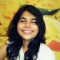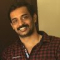Class Central is learner-supported. When you buy through links on our site, we may earn an affiliate commission.

# Mathematics for Machine Learning: Multivariate Calculus

### Overview

##### Class Central Tips
This course offers a brief introduction to the multivariate calculus required to build many common machine learning techniques. We start at the very beginning with a refresher on the “rise over run” formulation of a slope, before converting this to the formal definition of the gradient of a function. We then start to build up a set of tools for making calculus easier and faster. Next, we learn how to calculate vectors that point up hill on multidimensional surfaces and even put this into action using an interactive game. We take a look at how we can use calculus to build approximations to functions, as well as helping us to quantify how accurate we should expect those approximations to be. We also spend some time talking about where calculus comes up in the training of neural networks, before finally showing you how it is applied in linear regression models. This course is intended to offer an intuitive understanding of calculus, as well as the language necessary to look concepts up yourselves when you get stuck. Hopefully, without going into too much detail, you’ll still come away with the confidence to dive into some more focused machine learning courses in future.

### Syllabus

• What is calculus?
• Understanding calculus is central to understanding machine learning! You can think of calculus as simply a set of tools for analysing the relationship between functions and their inputs. Often, in machine learning, we are trying to find the inputs which enable a function to best match the data. We start this module from the basics, by recalling what a function is and where we might encounter one. Following this, we talk about the how, when sketching a function on a graph, the slope describes the rate of change of the output with respect to an input. Using this visual intuition we next derive a robust mathematical definition of a derivative, which we then use to differentiate some interesting functions. Finally, by studying a few examples, we develop four handy time saving rules that enable us to speed up differentiation for many common scenarios.
• Multivariate calculus
• Building on the foundations of the previous module, we now generalise our calculus tools to handle multivariable systems. This means we can take a function with multiple inputs and determine the influence of each of them separately. It would not be unusual for a machine learning method to require the analysis of a function with thousands of inputs, so we will also introduce the linear algebra structures necessary for storing the results of our multivariate calculus analysis in an orderly fashion.
• Multivariate chain rule and its applications
• Having seen that multivariate calculus is really no more complicated than the univariate case, we now focus on applications of the chain rule. Neural networks are one of the most popular and successful conceptual structures in machine learning. They are build up from a connected web of neurons and inspired by the structure of biological brains. The behaviour of each neuron is influenced by a set of control parameters, each of which needs to be optimised to best fit the data. The multivariate chain rule can be used to calculate the influence of each parameter of the networks, allow them to be updated during training.
• Taylor series and linearisation
• The Taylor series is a method for re-expressing functions as polynomial series. This approach is the rational behind the use of simple linear approximations to complicated functions. In this module, we will derive the formal expression for the univariate Taylor series and discuss some important consequences of this result relevant to machine learning. Finally, we will discuss the multivariate case and see how the Jacobian and the Hessian come in to play.
• Intro to optimisation
• If we want to find the minimum and maximum points of a function then we can use multivariate calculus to do this, say to optimise the parameters (the space) of a function to fit some data. First we’ll do this in one dimension and use the gradient to give us estimates of where the zero points of that function are, and then iterate in the Newton-Raphson method. Then we’ll extend the idea to multiple dimensions by finding the gradient vector, Grad, which is the vector of the Jacobian. This will then let us find our way to the minima and maxima in what is called the gradient descent method. We’ll then take a moment to use Grad to find the minima and maxima along a constraint in the space, which is the Lagrange multipliers method.
• Regression
• In order to optimise the fitting parameters of a fitting function to the best fit for some data, we need a way to define how good our fit is. This goodness of fit is called chi-squared, which we’ll first apply to fitting a straight line - linear regression. Then we’ll look at how to optimise our fitting function using chi-squared in the general case using the gradient descent method. Finally, we’ll look at how to do this easily in Python in just a few lines of code, which will wrap up the course.

### Taught by

Samuel J. Cooper, David Dye and A. Freddie Page

## Reviews

4.9 rating, based on 9 Class Central reviews

4.7 rating at Coursera based on 5494 ratings

Start your review of Mathematics for Machine Learning: Multivariate Calculus

•Shivani Sharma
The course is a great introduction to how one can translate pre-learned mathematical concepts into machine learning. I think it just makes you appreciate complicated mathematical equations as they are tied into neat computational applications.
For those who want an introduction to the math first, the course has plenty of explanatory videos as well. But as someone who did know the math, it just made me realize that my college math can actually be used to do something useful.
• This is the best course I have done so far, the practical part of the course is wonderful, you get to program a neural network just using numpy as a help, learn to differentiate, jacobians, hessians, newton ramphson, it is a very difficult course but it compensates when you can finish it.
• Anonymous
The teaching is clear and concise with an impressive breadth of material covered during the 6 weeks. There is an emphasis on developing intuition, and content is made highly engaging through visual descriptions of calculus techniques. Having completed the course, the understanding you are left with feels profound and rigorous.
• Anonymous
Clear explanations, cool animations, informative interactive activities and challenging assignments.
I especially enjoyed understanding back propagation from first principals and had also never seen multivariate Taylor series before!
• Anonymous
The part taught by Dr. Samuel J. Cooper is the best course I have ever seen in Calculus. It is very important to illustrate the essence of the topic with the methods used to solve the problems.
•Sajil C K
This course is an excellent one. It helped me grasp many complex concepts in an easy way. I hope every explanation/book/video follow this style. I strongly recommend this course to anyone.
• Anonymous
The concepts taught in the 3 courses are very relevant to Machine Learning. Professors Dye, Cooper and Deisenroth are excellent at teaching and making the material easy to understand. They make the best use of audiovisual technology I have seen in all online classes that I have taken.
I have continued to pursue machine learning education, with the mathematical foundation from these courses, it is much easier to understand how machines learn, and how to improve the performance of existing Machine Learning frameworks by proper choice of architecture and hyper-parameter tuning.

I strongly recommend starting your Machine Learning education by completing this certification.